# Split Dates into Separate Cells in Excel

This post will guide you how to split date and time into separate cells in Excel. How do I Separate dates and times into two separate cells in Excel. How to split a data into multiple cells in Excel. How to separate Date and time data into 2 different cells with a formula in Excel.

## Split Dates into Separate Cells

Assuming that you have a list of data in a range B1:B4 that contain date and time data, and you want to split those date and time into two separate cells in Excel. How to achieve it. You just need to do the following steps:

#1 Select one range of cells that you want to put the date into it.

#2 right click on it, and select Format Cells from the popup menu list. And the Format Cells dialog will open.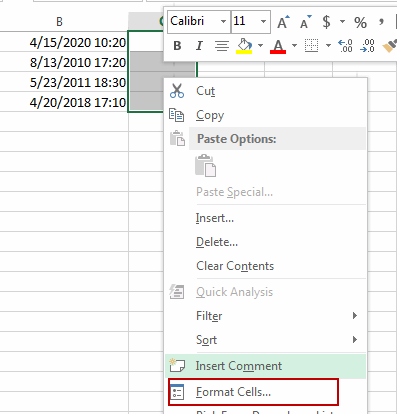#3 switch to Number tab, select Date in the Category list box, and select one type as you need. Click OK button.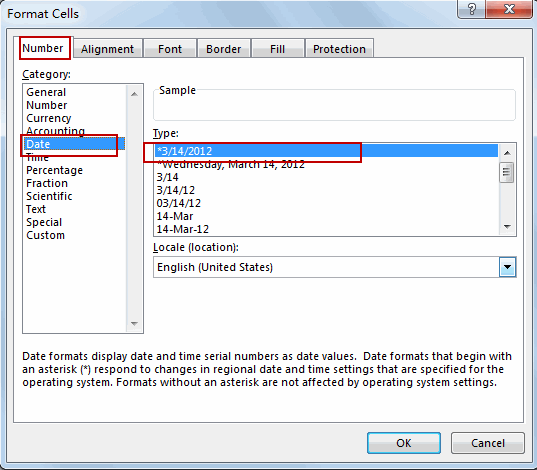#4 select another range of cells that you want to put the time into it.

#5 right click on it, and select Format Cells from the popup menu list. And the Format Cells dialog will open. And select Time in the Category list box, and select one time type as you need. Click OK button.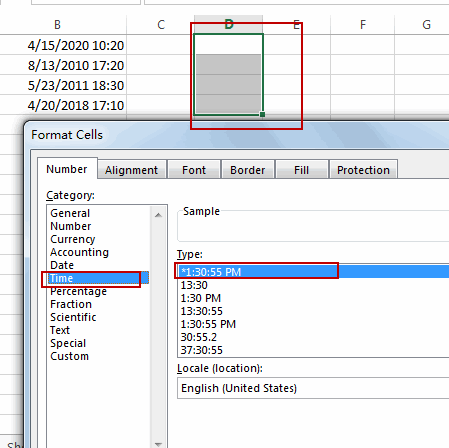#6 type the following formula into the date range, and then press Enter key, and drag the AutoFill Handle over other cells.

`=INT(B1)`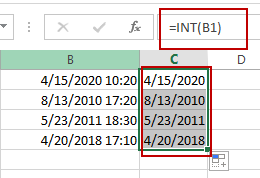#7 type the following formula into the time range, and press Enter key, and then drag the AutoFill Handle over other cells.

`=B1-C1`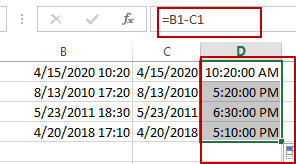### Related Functions

• Excel INT function
The Excel INT function returns the integer portion of a given number. And it will rounds a given number down to the nearest integer.The syntax of the INT function is as below:= INT (number)…

Related Posts
VLOOKUP – Retrieve Data from Another Worksheet

VLOOKUP is one of the key functions among all lookup & reference functions in Excel. It can scan and retrieve data from a static or dynamic table based on your lookup value. It can perform approximate match or exact match ...

Case Sensitive Lookup with SUMPRODUCT and EXACT

Today, we will show you how to use SUMPRODUCT and EXACT to perform a case sensitive exact match. In this article, we provide a simple example to calculate bonus for employees whose names are case-sensitive. If you meet similar scenarios ...

Basic Usage of INDEX & MATCH – Case Sensitive Lookup

In Excel, INDEX function and MATCH function are often used together for retrieving data from a particular position. MATCH function is one of Excel lookup & reference functions that can perform approximate match or exact match by setting different match ...

How to Auto Fill Weekdays or Weekends in Excel

Sometimes we may want to enter a sequence of days in excel for some purpose, and we can press Ctrl+; to insert current date into cell, and then drag the cell down to attach following days into other cells, then ...

How to Stack Data from Multiple Columns into One Column in Excel

In previous article, I have shown you the method to split data from one long column to multiple columns by VBA and Index function. This time if we want to stack data from multiple columns to one column, how can ...

How to Calculate Retirement Date and Remaining Years refer to Birth Date in Excel

Every company has its own police about the retirement date for employees. Suppose employees will be retired from a certain age of 60, how can we calculate the retirement date for everyone base on their birthdays? Actually, there are several ...

How to List Only Whole Numbers From Decimal Numbers in Excel

This post will guide you how to filter only whole numbers from decimal numbers in a list in Excel. How do I filter cells with whole numbers or non-whole numbers in Excel. List Only Whole Numbers Assuming that you have ...

How to Calculate Number of Weekends between Two Dates in Excel

This post will guide you how to count the number of weekends between two given dates in Excel. How to find out how many weekends are between two dates in Excel. How to count the number of Sundays between two ...

How to Check If a Number is Integer in Excel

This post will guide you how to check if a number is integer in Cells in Excel. How do I test if Cell value is integer with a formula in Excel. Check If Value is Integer Assuming that you have ...

How to Delete Numbers after Decimal Point in Excel

This post will guide you how to remove decimals without rounding in Excel. How do I delete numbers after decimal point with a formula in the given cell in Excel. Deleting Numbers after Decimal Point Assuming that you have a ...

Sidebar